# Algorithm

An algorithm is a sequence of well-defined steps that defines an abstract solution to a problem. Use this tag when your issue is related to algorithm design.1593347004

## A greedy algorithm is a simple

The Greedy Method is an approach for solving certain types of optimization problems. The greedy algorithm chooses the optimum result at each stage. While this works the majority of the times, there are numerous examples where the greedy approach is not the correct approach. For example, let’s say that you’re taking the greedy algorithm approach to earning money at a certain point in your life. You graduate high school and have two options:

#computer-science #algorithms #developer #programming #greedy-algorithms #algorithms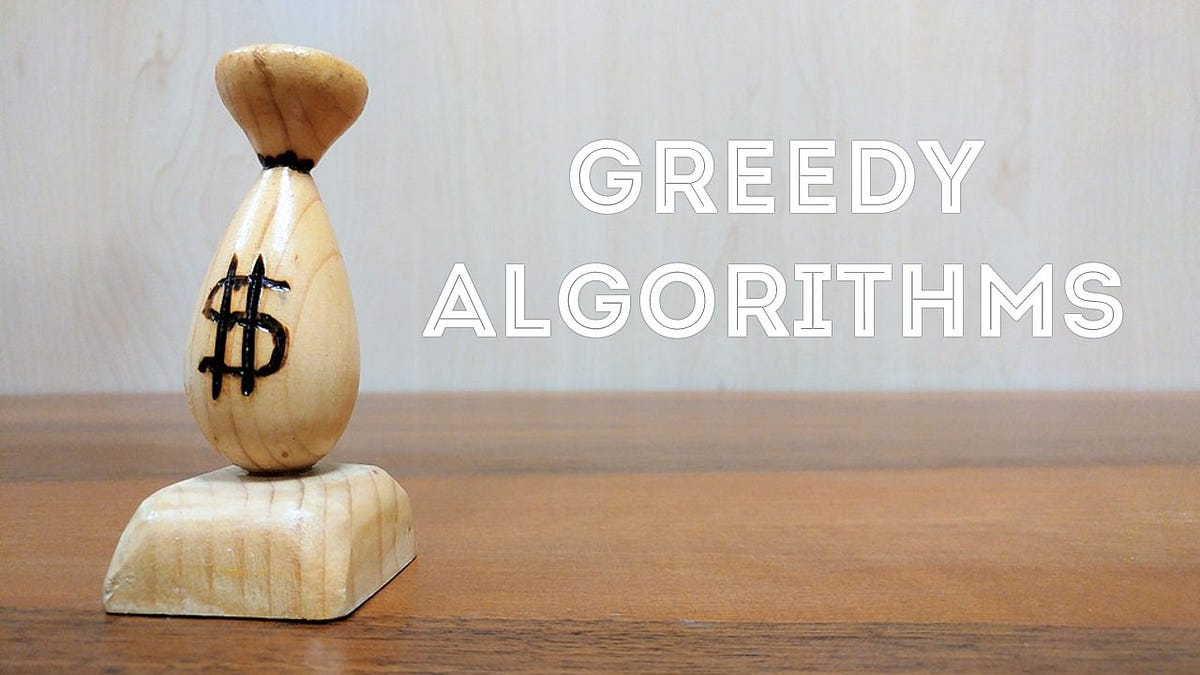1596427800

## KMP — Pattern Matching Algorithm

Finding a certain piece of text inside a document represents an important feature nowadays. This is widely used in many practical things that we regularly do in our everyday lives, such as searching for something on Google or even plagiarism. In small texts, the algorithm used for pattern matching doesn’t require a certain complexity to behave well. However, big processes like searching the word ‘cake’ in a 300 pages book can take a lot of time if a naive algorithm is used.

# The naive algorithm

Before, talking about KMP, we should analyze the inefficient approach for finding a sequence of characters into a text. This algorithm slides over the text one by one to check for a match. The complexity provided by this solution is O (m * (n — m + 1)), where m is the length of the pattern and n the length of the text.

Find all the occurrences of string pat in string txt (naive algorithm).

``````#include <iostream>
#include <string>
#include <algorithm>
using namespace std;

string pat = "ABA"; // the pattern
string txt = "CABBCABABAB"; // the text in which we are searching

bool checkForPattern(int index, int patLength) {
int i;
// checks if characters from pat are different from those in txt
for(i = 0; i < patLength; i++) {
if(txt[index + i] != pat[i]) {
return false;
}
}
return true;
}

void findPattern() {
int patternLength = pat.size();
int textLength = txt.size();

for(int i = 0; i <= textLength - patternLength; i++) {
// check for every index if there is a match
if(checkForPattern(i,patternLength)) {
cout << "Pattern at index " << i << "\n";
}
}

}

int main()
{
findPattern();
return 0;
}
view raw
main6.cpp hosted with ❤ by GitHub
``````

# KMP approach

This algorithm is based on a degenerating property that uses the fact that our pattern has some sub-patterns appearing more than once. This approach is significantly improving our complexity to linear time. The idea is when we find a mismatch, we already know some of the characters in the next searching window. This way we save time by skip matching the characters that we already know will surely match. To know when to skip, we need to pre-process an auxiliary array prePos in our pattern. prePos will hold integer values that will tell us the count of characters to be jumped. This supporting array can be described as the longest proper prefix that is also a suffix.

#programming #data-science #coding #kmp-algorithm #algorithms #algorithms1624867080

## Algorithm trading backtest and optimization examplesAlgorithm trading backtest and optimization examples.

### xbtusd-vanila-market-making-backtest-hedge1593350760

## Genetic Algorithm (GA): A Simple and Intuitive Guide

Learn what are metaheuristics and why we use them sometimes instead of traditional optimization algorithms. Learn the metaheuristic Genetic Algorithm (GA) and how it works through a simple step by step guide.

#genetic-algorithm #algorithms #optimization #metaheuristics #data-science #algorithms1626429780

## What is Optimization?

• Making something better.
• Increase efficiency.

## Optimization problem

• A problem in which we have to find the values of inputs (also called solutions or decision variables) from all possible inputs in such a way that we get the “best” output values.
• Definition of “best”- Finding the values of inputs that result in a maximum or minimum of a function called the objective function.
• There can be multiple objective functions as well (depends on the problem).

## Optimization algorithm

An algorithm used to solve an optimization problem is called an optimization algorithm.

## Evolutionary Algorithms

Algorithms that simulate physical and/or biological behavior in nature to solve optimization problems.

## Genetic Algorithm (GA)

• It is a subset of evolutionary algorithms that simulates/models Genetics and Evolution (biological behavior) to optimize a highly complex function.
• A highly complex function can be:
• 1. Very difficult to model mathematically.
• 2. Computationally expensive to solve. Eg. NP-hard problems.
• 3. Involves a large number of parameters.

## Background of GA

• Introduced by Prof. John Holland in 1965.
• The first article on GA was published in 1975.
• GA is based on two fundamental biological processes:
• 1. Genetics (by G.J. Mendel in 1865): It is the branch of biology that deals with the study of genes, gene variation, and heredity.
• 2. Evolution (by C. Darwin in 1875): It is the process by which the population of organisms changes over generations.

## Natural selection in Evolution

1. A population of individuals exists in an environment with limited resources.
2. Competition for those resources causes the selection of those fitter individuals that are better adapted to the environment.
3. These individuals act as seeds for the generation of new individuals through recombination and mutation.
4. Evolved new individuals act as initial population and Steps 1 to 3 are repeated.

## Applications of GA

• 1. Acoustics
• 2. Aerospace Engineering
• 3. Financial Markets
• 4. Geophysics
• 5. Materials Engineering
• 6. Routing and Scheduling
• 7. Systems Engineering

## Problems with GA

• 1. Population Selection Problem
• 2. Defining Fitness Function
• 3. Premature or rapid convergence of GA
• 4. Convergence to Local Optima

#evolutionary-algorithms #data-science #genetic-algorithm #algorithm1603767600

## Search Algorithms

Today, let us touch base on some fundamental concepts like search algorithms.

In simple terms, **searching **is a process of looking up a particular data record in the database or in the collection of items. A search typically answers as true or false whether the particular data in which we are referring is found or not and perform the next action accordingly.

Commonly used algorithms for search are:

• Linear search
• Binary search
• Interpolation search

Let us understand them in detail with some example

## Linear Search Algorithm

Linear Search Algorithm is the simplest and easiest form of the search algorithm. In this algorithm, a sequential search is made over all the items one by one to search for the targeted item. Each item is checked in sequence until the match is found. If the match is found, the searched item is returned otherwise the search continues till the end.

To make it easy to understand, let us see understand linear search using a flow diagram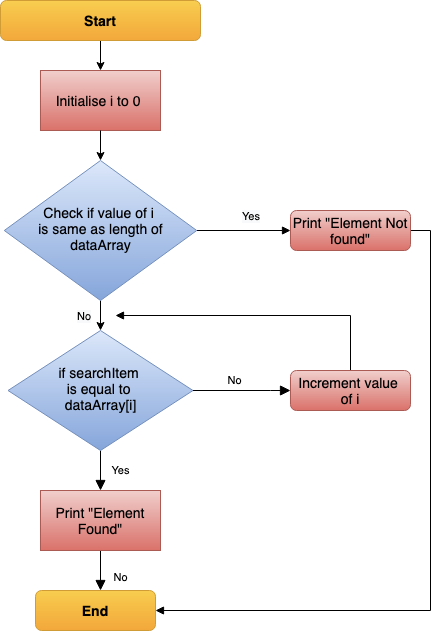Linear Search — Data Flow

### Points to note:

• Does not need sorted array list
• Performs equality comparisons
• The time complexity is O(n)
• Time taken to search elements keeps increasing as the number of elements is increased.

## Binary Search Algorithm

In _Binary search algorithm, _begins with an interval covering the whole array and diving it in half. If the value of the search key is less than the item in the middle of the interval, narrow the interval to the lower half. Otherwise narrow it to the upper half. Repeatedly check until the value is found or the interval is empty.

To make it easy to understand, let us see understand binary search using flow diagram and example as below.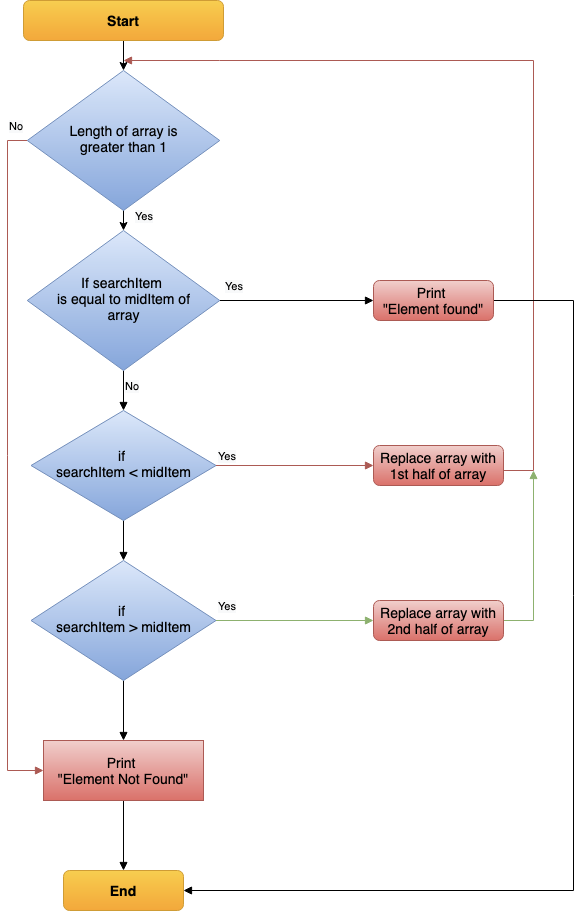Binary Search — Data Flow

### Points to note:

• The array needs to be sorted
• Performs ordering comparisons
• Time complexity to O(log n).
• Search is done to either half of the given list, thus cut down your search to half time

#sorting-algorithms #algorithms #data-structures #search-and-sort #searching-algorithm1626421931

## Coin Change Problem Using Greedy Algorithm

In this post, we will look at the solution for Coin Change Problem using Greedy Algorithm.

But before that, let’s understand what Greedy Algorithms are in the first place.

### 1 - What are Greedy Algorithms?

Greedy Algorithms  are basically a group of algorithms to solve certain types of problems. The key part about greedy algorithms is that they try to solve the problem by always making a choice that looks best for the moment .

### 2 - Introducing the Coin Change Problem

The famous coin change problem is a classic example of using greedy algorithms.

### 3 - Coin Change Problem Greedy Approach Implementation

Below is an implementation of the above algorithm using C++. However, you can use any programming language of your choice.

### 4 - Issue with Greedy Algorithm Approach

While the coin change problem can be solved using the Greedy algorithm, there are scenarios in which it does not produce an optimal result.

#tutorial #algorithm #data structure #algorithm analysis #greedy algorithm1594453947

## Basic AI Algorithms

To solve a problem with a computer, it is necessary to represent the problem in numerical or symbolic form and offer a specific procedure using a programming language. However, working with problem-solving in the artificial intelligence (AI) field, it is difficult to specify a formulation of a problem from the beginning. Therefore, the flexibility of choosing a solution procedure during the observation of state changes is highly required. Some problems can be represented by a graph or tree structure where each node represents a state of the problem. This kind of problem formulation method is called State-space representation.

## State-space representation

In a state-space representation method, each state of the problem is represented by a node of a graph or tree structure. In this case, the problem is translated as a search problem to determine the goal under specific operators and restrains. In this post, I will introduce Traveling Salesman Problem (TSP) as an example.

Representation a problem with the state-space representation needs:

(1). A set of states of the problem

(2). A set of operators to operate between states of the problem

(3). Initial state and final state(goal)

### Traveling Salesman Problem (TSP)

Consider the following situation. You are given a list of n cities with the distance between any two cities. Now, you have to start with your office and to visit all the cities only once each and return to your office. What is the shortest path can you take? This problem is called the Traveling Salesman Problem (TSP).

#search-algorithm #travelingsalesmanproblem #a-algorithm #ai-algorithms #heuristic-evaluation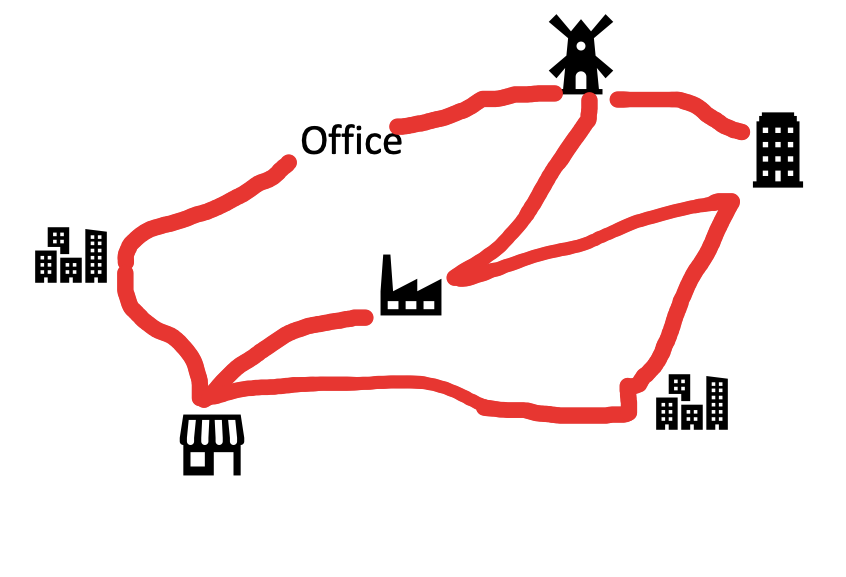1598518020

## Let Us Understand Searching Algorithms

When searching for data, the difference between a fast application and a slower one lies in the accurate use of search algorithm. Searching algorithms is a basic, fundamental step in computing done via step-by-step method to locate a specific data among a collection of data.

All search algorithms make use of a search key in order to complete the procedure. And they are expected to return a success or a failure status ( in boolean true or false value).

In computer science, there are various type of search algorithms available and the way they are used decides the performance and efficiency of the data available( the manner in which the data is being used).

What is a Search Algorithm?

According to Wikipedia- Search algorithm is-

Any algorithm which solves the search problem, namely, to retrieve information stored within some data structure, or calculated in the search space of a problem domain, either with discrete or continuous values.

Searching Algorithms are designed to check or retrieve an element from any data structure where it is being stored.

They search for a target (key) in the search space, like-

1. All students in the class.
2. All numbers in a given list.

#data structure #algorithm skills #algorithms #searching algorithms #big data1597773900

## A Survey on Shuffled Frog-Leaping Algorithm

### Nature; The most excellent teacher of humankind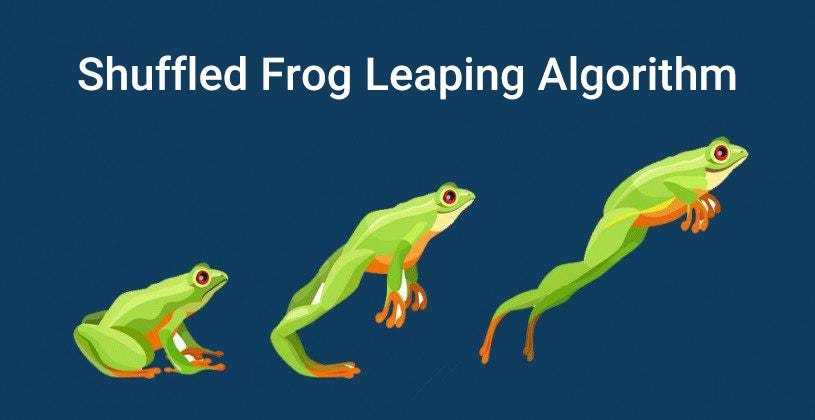The Shuffled Frog Leaping Algorithm (SFLA) is one of the most innovative optimization algorithms inspired by the social behavior of frogs in nature, and in terms of classification, among behavioral algorithms or Memetic Algorithms are included. Other names for the frog jump optimization algorithms include the frog jump algorithm, frog leaping algorithm, and SFLA algorithm. This algorithm was first proposed by Eusuff and Lansey in 2003, although many papers have since been submitted to improve this algorithm. At the bottom of the download link is the main article of the frog optimization algorithm.

## What is the SFLA algorithm?

The SFLA algorithm is an algorithm based on Metaheuristic memetics. The memetic algorithm is a population-based algorithm used for complex and significant optimization problems. The main idea of ​​this algorithm is to use a local search method within the structure of the genetic algorithm to improve the aqueous performance of the search intensification process. The memetic algorithm first encrypts the sum of the initial answers, then Ibn algorithm calculates the utility of each response based on a fitness function and generates new solutions.

The SFLA algorithm is inspired by how frogs search for food. This algorithm uses the Nomometric method to search locally among subgroups of frogs. The frog hybrid jump algorithm uses the hybrid strategy and allows the exchange of messages in local search. This algorithm combines the advantages of the Nomometric algorithm and particle group optimization. In the frog hybrid jump algorithm, words are exchanged not only in local search but also in global search. Thus, Local and Global searches are well combined in this algorithm. The frog hybrid jump algorithm is highly searchable and easy to implement. The frog hybrid jump algorithm can solve many nonlinear, undetectable, and multi-state problems.

#machine-learning #towards-data-science #artificial-intelligence #evolutionary-algorithms #optimization-algorithms #algorithms1593362160

## Validate the Correctness of an Evolutionary Optimization Algorithm

1. Introduction
2. Correctness Validation
3. A Brief Discussion on Performance Evaluation
4. Conclusion
5. References
6. Some Related Medium Posts

# 1. Introduction

Evolutionary and Metaheuristic optimization algorithms are considered as a subset of Artificial Intelligence (AI). These algorithms try to imitate nature mechanisms and solve an optimization problem. For example, the Genetic Algorithm (GA) uses inspired biological mechanisms such as reproduction, cross-over, selection, mutation, and recombination to find the best solution for an optimization problem. Another example can be an Imperialist Competitive Algorithm (ICA) where social mechanisms like domination, revolution, and colonization are used to find a solution . Evolutionary algorithms are usually performing well in complex optimization problems where using classical approaches is mathematically difficult. They can easily deal with nonlinear problems without being stuck in local minimums.

To optimize a problem with an evolutionary optimization algorithm one can use a certified toolbox. However, for some algorithms, either there is no available certified toolbox or one may prefer to implement his/her own code with any program languages. In this situation, the most important questions are — How we can trust the written code? How we can validate the correctness of our implemented evolutionary or metaheuristic code?

In this post, some of the existing ways to check and validate the correctness of an implemented evolutionary optimization algorithm have been addressed briefly. In addition, a discussion regarding the performance evaluation of optimization algorithms is provided using an example of Ackley function.

# 2. Correctness Validation

There are different ways to check the correctness and accuracy of an implemented metaheuristic multi-objective optimization algorithm (e.g. Genetic Algorithm (GA) or Differential Evolution (DE)). The following approaches can be addressed briefly to check the correctness of the implemented optimization algorithm:

A) Comparison with classical approaches: In some problems, it can be possible to run both the classical approach and multi-variate GA to make sure the results are correct and accurate. However, in most cases, because of mathematical complexity using a classical approach for comparison is not possible.

B) Using Well-known Benchmarks: The correctness of the code can be also proven though existing benchmarks. The used algorithm can be validated via well-known benchmarks [2–6].

C) Using Expert Idea: An expert of the system can check the results and their rationality. They should all agree that the final results are logical. If for a problem exact minimum cost value is needed, then using an expert idea would not be convincing.

D) Convergence: The convergence of the implemented multi-objective genetic algorithm can be easily observed through the plot of cost vs. iterations. There are also other factors such as roundoff errors and local extrema that have the same perspective.

E) Domination Factor: The new generation of optimization algorithms such as NSGA-II, NSGA-III, and MOPSO usually have a function to separate dominant results from non-dominant one. So, all final results should be dominant and with a proper initial population, it is very rare to be trapped by a local minimum. However, there is no guarantee that they find the global minimum of the system. For more information regarding the domination please check .

#metaheuristics #artificial-intelligence #evolutionary-algorithms #optimization-algorithms #performance #algorithms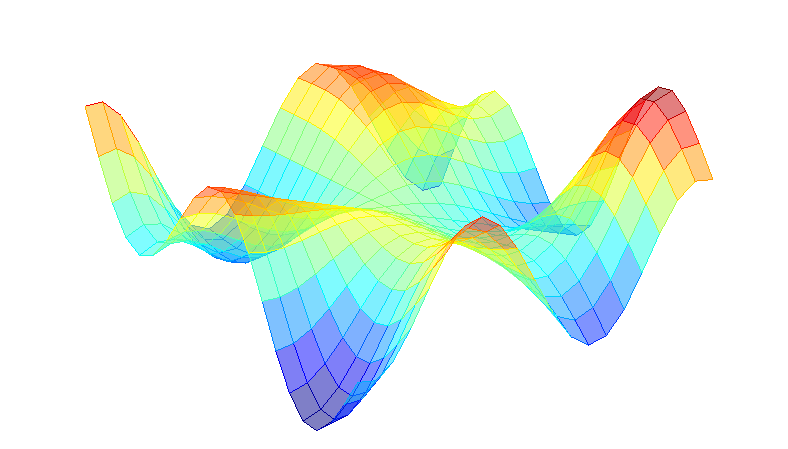1624457760

## Code and notes for the Algorithms Specialization course

### Stanford Algorithms Specialization

Code and notes for the Algorithms Specialization course offered by Stanford University.

A portion of the MergeSort algorithm:

#algorithms #algorithms specialization #specialized algorithms1623683340

## How I Made a 65% ROI with this Boeing Trading Algorithm

Since the market crashed in March of 2020 the rebound has been swift and irrational.

Boeing, for example, is in many ways worse off than it was in March.

It’s clear air travel has plummeted and that airlines will be impacted for

years. Where will airlines get the money to purchase planes?

An example of one headline in April: “Boeing customers cancel staggering 150 Max plane orders”.

One thing I’ve noticed is that since the end of March you can

basically just buy every dip and expect a pop, selling the next day. I

mentioned this to a friend on Friday and decided to backtest it.

Well, sure enough it works!

I’ll mention I made one modification. Originally I wrote the system like so:

1. Check if Boeing is down more than 3% 15 minutes from close

2. If yes, buy with 100% of portfolio

3. The next day, 15 minutes from open liquidate the portfolio.

This worked OK. Great actually! It returned about 25%. But want to know what really kicked it up a notch?

Instead of just selling the next day, I only sell if the position is

sitting at a realized gain. So e.g. if the next day its flat or drops

another 1%, don’t sell it, just keep holding on until its up and THEN

sell. Of course, this is completely insane and you would have to expect

the market to only go up, but that’s what has been happening.

Guess what? This simple system returned a whopping 65% in two-ish months. Yeah, I know, crazy.

Check out the backtest screenshot:

And here are the raw trading logs for those that want to see the dates the trades were made:

### Here is the code!

Before we look at the code, I’ll just mention here are the details of the backtest:

• start at April first and go until last Friday (June 19th 2020)

• end up with about 165k or a 65% return.

I wrote this little script on Quant Connect. The screenshot at the

top of the page is the backtest result, and the code below is everything you need to try this out.

Note the place I mentioned in the code you should comment if you want this to be a little bit less insane.1593358380

## A genetic algorithm is a higher level method to produce

• A genetic algorithm (GA) is a higher level method to produce/ generate a sufficiently good solution to an optimization problem, inspired by the process of natural selection that belongs to the larger class of evolutionary algorithms (EA).
• Genetic algorithms are commonly used to generate high-quality solutions to optimization and search problems by relying on biologically inspired operators such as mutation, crossover and selection.
• John Holland introduced genetic algorithms in 1960 based on the concept of Darwin’s theory of evolution

Evolutionary algorithms (EA):

In artificial intelligence (AI), an evolutionary algorithm (EA) is a subset of evolutionary computation, a generic population-based metaheuristic optimization algorithm. An EA uses mechanisms inspired by biological evolution, such as reproduction, mutation, recombination, and selection.

Evolutionary algorithms have three main characteristics:

· Population-Based: Evolutionary algorithms are to optimize a process in which current solutions are bad to generate new better solutions. The set of current solutions from which new solutions are to be generated is called the population.

· Fitness-Oriented: If there are some several solutions, how to say that one solution is better than another? There is a fitness value associated with each individual solution calculated from a fitness function. Such fitness value reflects how good the solution is.

· Variation-Driven: If there is no acceptable solution in the current population according to the fitness function calculated from each individual, we should make something to generate new better solutions. As a result, individual solutions will undergo a number of variations to generate new solutions.

In

a genetic algorithm, a population of candidate solutions (called individuals, creatures, or phenotypes) to an optimization problem is evolved toward better solutions. Each candidate solution has a set of properties (its chromosomes or genotype) which can be mutated and altered; traditionally, solutions are represented in binary as strings of 0s and 1s, but other encodings are also possible.

The process of natural selection starts with the selection of fittest individuals from a population. They produce offspring which inherit the characteristics of the parents and will be added to the next generation. If parents have better fitness, their offspring will be better than parents and have a better chance at surviving. This process keeps on iterating and at the end, a generation with the fittest individuals will be found.

Six phases are considered in a genetic algorithm:

· Initial population

· Fitness function

· Selection

· Crossover

· Mutation

· Termination

flowchart for process in a genetic algorithm

#evolutionary-algorithms #optimization #genetic-algorithm #machine-learning #ai #algorithms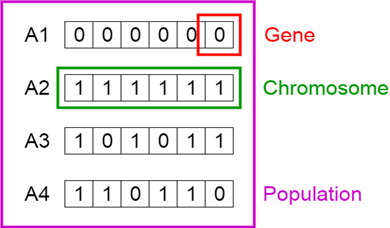1624388400

## Python Algorithms for Interviews. DO NOT MISS!!!

Learn about common algorithm concepts in Python and how to solve algorithm challenges you may encounter in an interview.

⭐️Contents⭐️
⌨️ (0:00:00) Big O Notation
⌨️ (0:22:08) Big O Examples
⌨️ (0:43:01) Array Sequences
⌨️ (0:53:23) Dynamic Arrays
⌨️ (1:06:26) Array Algorithms
⌨️ (1:20:40) Largest Sum
⌨️ (1:31:27) How to Reverse a String
⌨️ (1:57:32) Array Analysis
⌨️ (2:00:00) Array Common Elements
⌨️ (2:28:54) Minesweeper
⌨️ (3:08:16) Frequent Count
⌨️ (3:16:58) Unique Characters in Strings
⌨️ (3:28:35) Non-Repeat Elements in Array

📺 The video in this post was made by freeCodeCamp.org
The origin of the article: https://www.youtube.com/watch?v=p65AHm9MX80&list=PLWKjhJtqVAbnqBxcdjVGgT3uVR10bzTEB&index=8
🔥 If you’re a beginner. I believe the article below will be useful to you ☞ What You Should Know Before Investing in Cryptocurrency - For Beginner
⭐ ⭐ ⭐The project is of interest to the community. Join to Get free ‘GEEK coin’ (GEEKCASH coin)!